# KVM

CSAW'18 CTF Qualification - RE (500 pts).

# CSAW’18 CTF Qualification: KVM

Event Challenge Category Points Solves
CSAW’18 CTF Qualification KVM Crackme 500 ¯\(ツ)

### TL;DR

This write-up deals with a crackme challenge based on KVM presented at CSAW’18 CTF Qualification. KVM (for Kernel-based Virtual Machine) is a full virtualization solution for Linux x86 hardware containing virtualization extensions (Intel VT or AMD-V).

The program runs a code in a virtual machine created with KVM which check the user input. The password is compressed with the Huffman coding.

### Inside the binary

You can find the associated file here : kvm. Because of the binary name, we would expect some code related with KVM. The first operation of binary is to open the device /dev/kvm.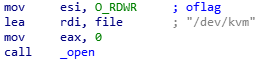To control specific device operations, the program uses the ioctl system call (DeviceIoControl is the equivalent on Windows).

``````int ioctl(int fd, unsigned long request, ...);
``````

Specific operations on kvm might be:

• get version
• create vm
• create vcpu
• run vm

After binary pass the file descriptor as argument of the ioctl system call.The operation associated with request code is 0x0000AE00 can be retrieved in kvm.h.

``````#define KVMIO 0xAE

/*
* ioctls for /dev/kvm fds:
*/
#define KVM_GET_API_VERSION _IO(KVMIO, 0x00)
#define KVM_CREATE_VM       _IO(KVMIO, 0x01) /* returns a VM fd */
``````

A request code is composed of

• NR: the command number (0x00)
• Type: magic number to identify the device (0xAE)
• Size: size of the command argument (0)
• Direction: read,write,both or none (0)
NR Type Size Direction
0 - 7 8 - 15 16 - 29 30 - 31

To sum up this piece of code retrieves the kvm version.

I look for the string “KVM_GET_API_VERSION” on google to find a minimal example of kvm usage in C. The following link https://github.com/dpw/kvm-hello-world contains code to run hello world program with KVM.

I retrieved key functions (vm_init,vcpu_init,run_protected_mode) from the hello world program.

I imported some useful structures, which will be useful for the future, in IDA .

``````struct vm {
int sys_fd;
int fd;
char *mem;
};
``````

vm structure represents the virtual machine:

• sys_fd is a file descriptor associated with /dev/kvm
• fd is a file descriptor associated with the virtual machine which is created in vm_init
• mem is a pointer to the memory (code and data) used by the virtual machine
``````struct vcpu {
int fd;
struct kvm_run *kvm_run;
};
``````

vcpu structure represents a virtual cpu of the virtual machine:

• fd is a file descriptor associated with the virtual cpu which is created in vcpu_init
• kvm_run is a structure used to communicate information about the CPU between the kernel and user space. In particular, whenever hardware virtualization stops (called a “vmexit”), the kvm_run structure will contain information about why it stopped.

Now we will search which piece of code is virtualized.

### Virtual machine code

The following assembly code copies some x86 code in memory allocated earlier.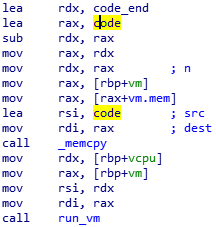#### Extracting code

code and code_end label are located at 0x202174 and 0x20348C respectively. We can easily extract code with python and disassemble it with IDA.

``````CODE_SIZE = 0x20348C - 0x202174
f = open("kvm","rb")
f.seek(0x2174)
code = f.read(CODE_SIZE)
f.close()

f = open("payload","wb")
f.write(code)
f.close()
``````

#### User input

The payload begins with a call to a unknown function. We can guess from the arguments that this function is a read(unsigned char* buffer,int size)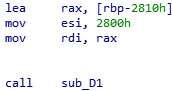If we look inside it, we can see that program uses the instruction in al,dx. IN copies the value from the I/O port specified by the second operand (dx) to the destination operand (al). I/O port is used to communicate with devices associated with the processor. Port 0xE9 is often used by some emulators to directly send text to the hosts console.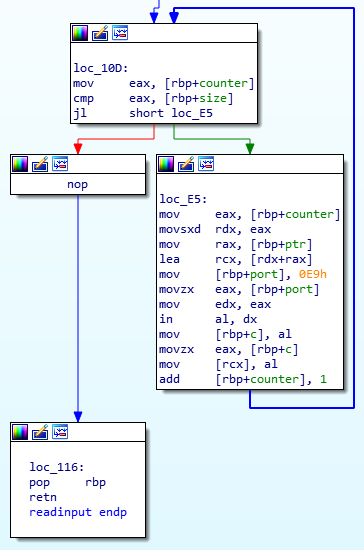When virtualized code performs a I/O operation, kvm stop the virtual machine and set KVM_EXIT_IO in exit_reason member of kvm_run structure.

In this case the program checks if the I/O port is equal to 0xE9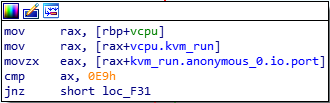Then it checks the direction (input or output) of the I/O operation.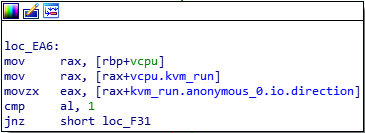According to the direction the program reads a character from standard input (stdin), or write a character on the standard output (stdout). So the virtualized code reads 0x2800 bytes from standard input.

#### Mysterious function

Then the program passes each character to a mysterious function. If the function returns FALSE (0) then the programm display “Wrong!” and exit.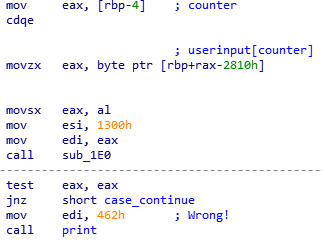The function takes another arguments which seems to be a pointer to a binary tree.

Node 1: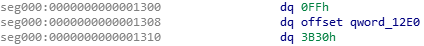Left child of node 1: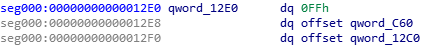The first element is the node value and it is followed by two pointers for:

• left node child
• right node child

A node value different from 0xFF indicates a leaf. The following code presents the C equivalent structure.

``````typedef struct node_t
{
uint64_t value;
uint64_t left;
uint64_t right;
}
``````

I wrote a simple python script to convert the binary tree in graphivz format and this is the partial result. When the node is a leaf the script display the value of the node after the address.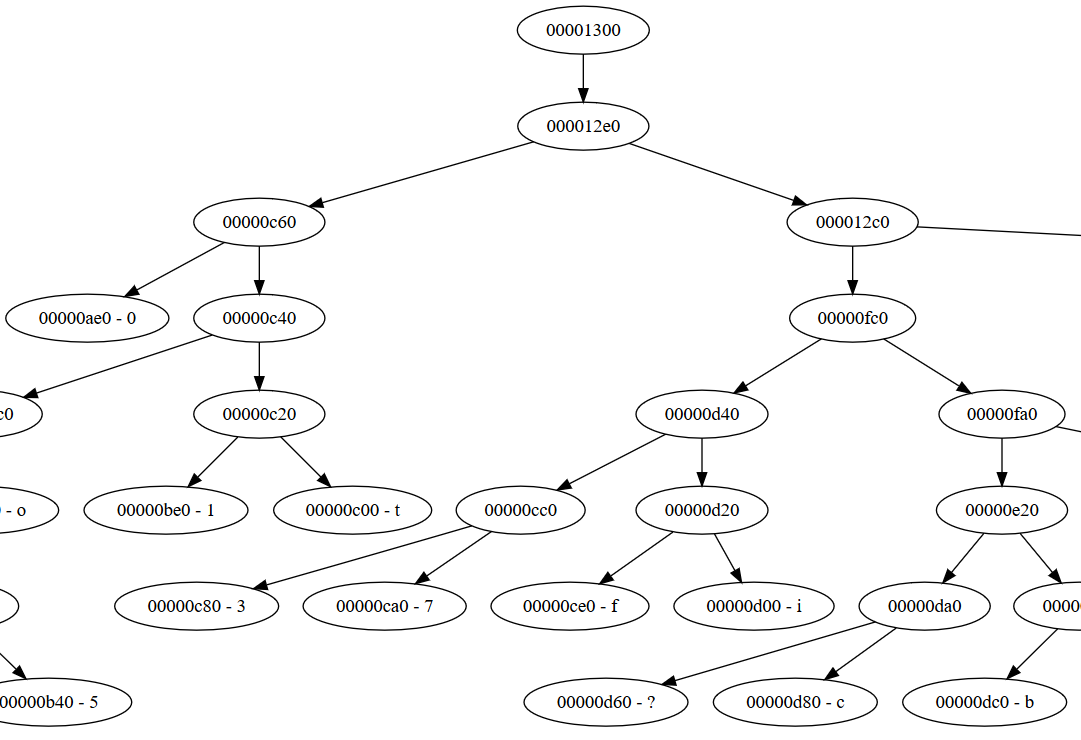### Obfuscated code

We have all the keys to discover what the function does, but the code seems obfuscated. As you can see there is a hlt instruction which stops the CPU. So the exit_reason member of kvm_run structure takes the value KVM_EXIT_HLT.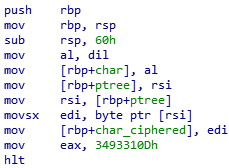The program handles this case, it calls ioctl with 0x8090AE81 (KVM_GET_REGS) as request code to get all registers values of the virtual CPU. The structure which represents cpu registers is of type kvm_regs.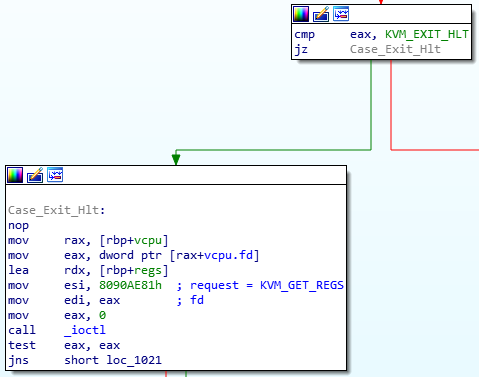The value of EAX register is passed to a function which return a new RIP for the virtual CPU.

In the following piece of code you can see that the program use eax after custom_kvm_jump call to set the RIP member in the kvm_regs structure. Then the program call ioctl with 0x4090AE82 (KVM_SET_REGS) as request code to set new register’ values for the virtual CPU.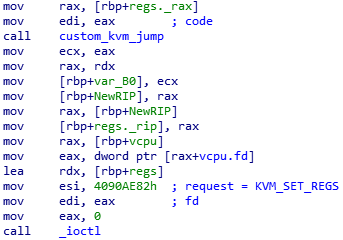If we look inside the function, the code search the RIP value in a table which contains 13 (TableSize) structure that I called jmp_t. It allows to associate a id (for example 0x3493310D) with a RIP value.

A jmp_t structure contains:

• id value placed in eax before hlt instruction
• reg_rip address to jump after hlt instruction
``````typedef struct jmp_t{
uint64_t id;
uint64_t reg_rip;
}
``````We look for the id 0x3493310D and we found that 0x32C is the associated value.So the cpu jump at address 0x32C. Then I desobfuscated all code and I translated the assembly code into corresponding C code. I realised that this C code is an implementation of the Huffman coding algorithm.

``````sub(char* c,node_t* root)
{
if(root->value == 0xFF)
{
ret = sub(c,root->left)
if(ret == 1)
{
writebit(0);
return 1;
}
else
{
ret = sub(c,root->right)
if(ret == 1)
writebit(1);
return 1;
else
return 0;
}
}else
{
if(c == root->value)
return 1;
else
return 0;
}
}
``````

The writebit function start writing bits at address 0x1300.

To sum up the binary compress the user input using Huffman coding and store the result at 0x1300. But where is the comparison ?

I found a function which takes three parameters the compressed input, some bytes, and a size. It looks like a memcmp. If memcmp return 0 (equal case) the binary print ‘Correct!’.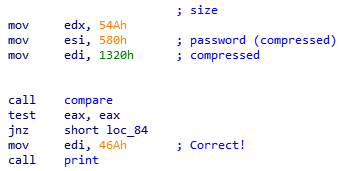### Scripting

We only have to decompress the password by using the Hoffman decoding algorithm with the correct tree.

The following class represents a node which has two children (left,right) and a value. The member id is the address of node structure in the payload file.

``````import struct

class Node():
def __init__(self,id,value):
self.id = id
self.value = value
self.left = None
self.right = None

def Name(self):
if self.value != 0xFF:
return "%08.8x - %c" % (self.id,chr(self.value))
else:
return "%08.8x" % (self.id)
``````

I wrote some utility function to construct a Node from a given address

``````
def ReadQword(f,addr):
f.seek(addr)
dword = struct.unpack("<Q",f.read(8))
return dword

def ReadNode(f,addr):
value = ReadQword(f,addr)
node = Node(addr,value)
if value != 0xFF:
pass
if value == 0xFF:
left_addr = ReadQword(f,addr+8)
right_addr = ReadQword(f,addr+16)
if left_addr != 0 and left_addr < 0x1310:
node.left = ReadNode(f,left_addr)
if right_addr != 0 and right_addr < 0x1310:
node.right = ReadNode(f,right_addr)
return node

f = open("payload.bin","rb")
tree = ReadNode(f,0x1300)
f.close()
``````

Then the script reads the compressed password and convert it into a bit streams.

``````f = open("payload.bin","rb")
f.seek(0x580)
data = f.read(0x54A)
binarystring =""
for c in data:
for i in range(0,8):
if (ord(c) & (1 << i)) == 0:
binarystring = "0" +binarystring
else:
binarystring = "1" + binarystring
``````

I iterate through the bit stream. To find a character corresponding to current bits, we use following simple steps:

• We start from root and do following until a leaf is found
• If current bit is 0, we move left node to the tree
• If the bit is 1, we move to right node of the tree
• If during traversal, we encounter a leaf node, we append this character to the decoded data and then again continue the iteration of the encoded data starting from step 1.
``````data=""
root = tree
currentnode = root
for b in binarystring:
if currentnode == root and b=="1":
continue
if b=="0":
currentnode = currentnode.left
elif b=="1":
currentnode = currentnode.right
if currentnode.value != 0xFF:
data = chr(currentnode.value)+data
currentnode = root
print(data)
``````

The script output the decompressed password result which is a tar archive containing the flag \o/ .

``````flag.txt0000664000175000017500000000007113346340766011602 0ustar  toshitoshiflag{who would win?  100 ctf teams or 1 obfuscat3d boi?}
``````

Tomtombinary